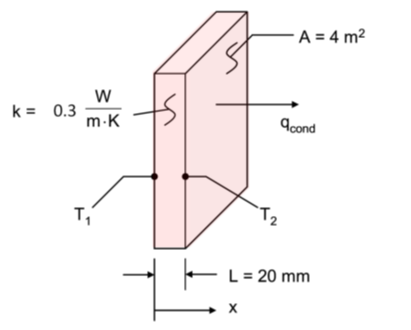## Find the Heat Transfer and Flux

The below rectangular sheet of rigid insulation has a thermal conductivity of k = 0.3 W/m∙K, and experiences a temperature difference of T1-T2=10K across its 20-mm-thick material face sheet.
1. What is the heat flux through a 2 m x 2 m sheet of the insulation?
2. What is the rate of heat transfer through the sheet of insulation?Hint
Heat flux is:
$$q_x"=-k\frac{dT}{dx}=k\frac{T_1-T_2}{L}$$$where $$k$$ is the thermal conductivity, $$dT$$ is the change in temperature, and $$dx$$ is the distance. Hint 2 Fourier’s Law of Conduction: $$q_x=q_x"\times A$$$
where $$q_x$$ is the rate of heat transfer, $$q_x"$$ is the heat flux, and $$A$$ is the area.
ASSUMPTIONS : (1) One-dimensional conduction in the x-direction, (2) Steady-state conditions, (3) Constant properties.

The heat flux equation is:
$$q_x"=-k\frac{dT}{dx}=k\frac{T_1-T_2}{L}$$$where $$k$$ is the thermal conductivity, $$dT$$ is the change in temperature, and $$dx$$ is the distance. Solving, $$q_x"=0.3\frac{W}{m\cdot K}\times \frac{10K}{0.02m}=150\: \frac{W}{m^2}$$$
Fourier’s Law of Conduction:
$$q_x=q_x"\times A$$$where $$q_x$$ is the rate of heat transfer, $$q_x"$$ is the heat flux, and $$A$$ is the area. Thus, the heat rate is: $$q_x=150\frac{W}{m^2}\times 4m^2=600\: W$$$
1. $$150\: \frac{W}{m^2}$$
2. $$600\:W$$